# Splitting a digraph with minimum outdegree constraints

 Importance: High ✭✭✭
 Author(s): Alon, Noga
 Subject: Graph Theory » Directed Graphs
 Keywords:
 Posted by: fhavet on: March 1st, 2013
Problem   Is there a minimum integer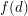such that the vertices of any digraph with minimum outdegreecan be partitioned into two classes so that the minimum outdegree of the subgraph induced by each class is at least?

Thomassen [T] proved the conjecture whenand showed. In fact, this case is equivalent to the case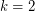of the Bermond-Thomassen Conjecture.

The existence of the corresponding functionfor the undirected analogue is easy and has been observed by many authors. Stiebitz [S] even proved the following tight result: if the minimum degree of an undirected graphis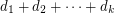, where each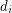is a non-negative integer, then the vertex set ofcan be partitioned intopairwise disjoint sets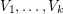, so that for all, the induced subgraph on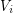has minimum degree at least. This is clearly tight, as shown by an appropriate complete graph.

## Bibliography

*[A] Noga Alon, Disjoint Directed Cycles, Journal of Combinatorial Theory, Series B, 68 (1996), no. 2, 167-178.

[S] M. Stiebitz, Decomposing graphs under degree constraints, J. Graph Theory 23 (1996), 31-324.

[T] C. Thomassen, Disjoint cycles in digraphs, Combinatorica 3 (1983), 393 - 396.

* indicates original appearance(s) of problem.